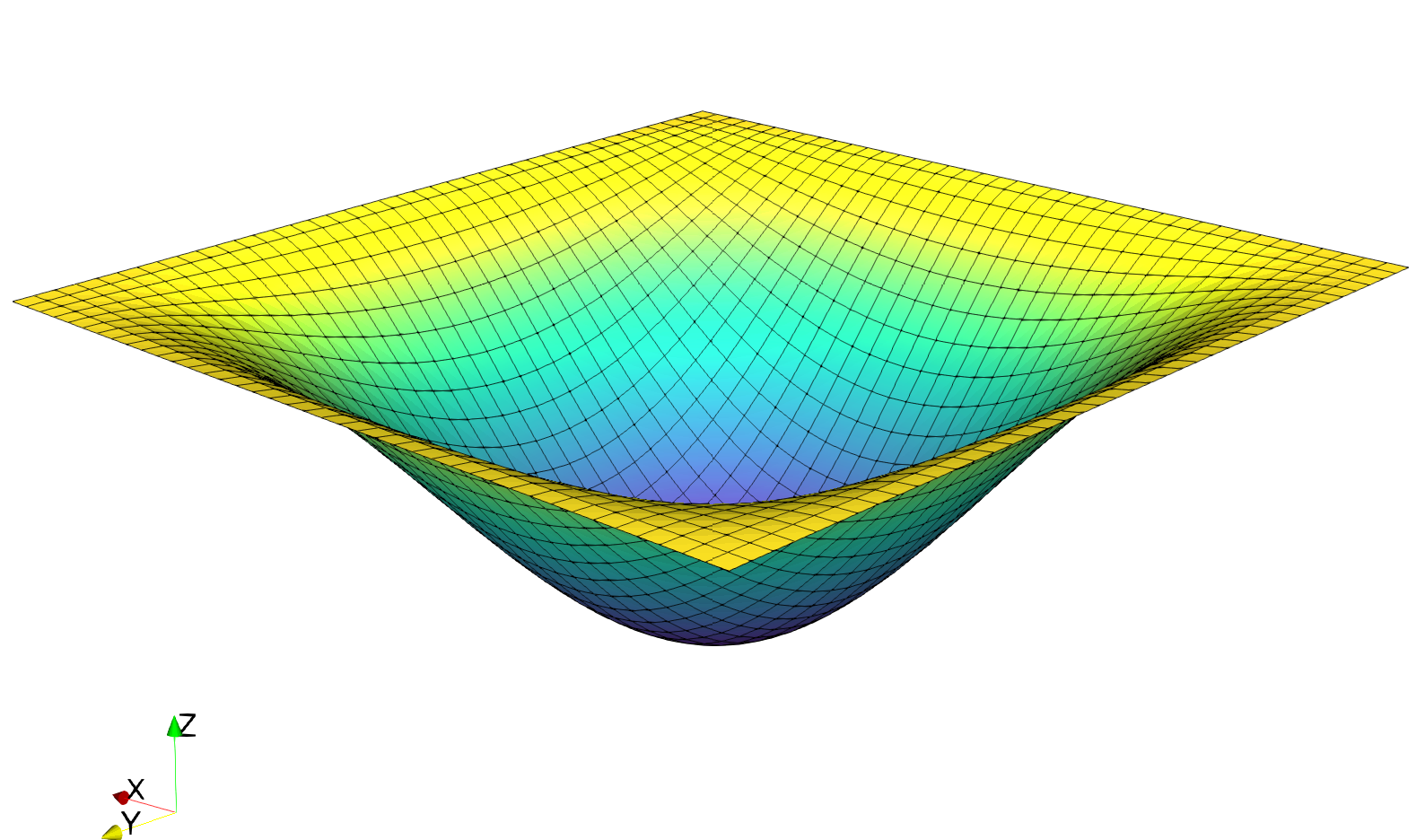Introduction¶

This program solves the Reissner-Mindlin plate equations on the unit square with uniform transverse loading and fully clamped boundary conditions. The corresponding file can be obtained from reissner_mindlin_quads.py.

It uses quadrilateral cells and selective reduced integration (SRI) to remove shear-locking issues in the thin plate limit. Both linear and quadratic interpolation are considered for the transverse deflection $$w$$ and rotation $$\underline{\theta}$$.

Note

Note that for a structured square grid such as this example, quadratic quadrangles will not exhibit shear locking because of the strong symmetry (similar to the criss-crossed configuration which does not lock). However, perturbating the mesh coordinates to generate skewed elements suffice to exhibit shear locking.

The solution for $$w$$ in this demo will look as follows:Implementation¶

Material parameters for isotropic linear elastic behavior are first defined:

from dolfin import *

E = Constant(1e3)
nu = Constant(0.3)

Plate bending stiffness $$D=\dfrac{Eh^3}{12(1-\nu^2)}$$ and shear stiffness $$F = \kappa Gh$$ with a shear correction factor $$\kappa = 5/6$$ for a homogeneous plate of thickness $$h$$:

thick = Constant(1e-3)
D = E*thick**3/(1-nu**2)/12.
F = E/2/(1+nu)*thick*5./6.

The uniform loading $$f$$ is scaled by the plate thickness so that the deflection converges to a constant value in the thin plate Love-Kirchhoff limit:

f = Constant(-thick**3)

The unit square mesh is divided in $$N\times N$$ quadrilaterals:

N = 50

Continuous interpolation using of degree $$d=\texttt{deg}$$ is chosen for both deflection and rotation:

deg = 1
We = FiniteElement("Lagrange", mesh.ufl_cell(), deg)
Te = VectorElement("Lagrange", mesh.ufl_cell(), deg)
V = FunctionSpace(mesh, MixedElement([We, Te]))

Clamped boundary conditions on the lateral boundary are defined as:

def border(x, on_boundary):
return on_boundary

bc =  [DirichletBC(V, Constant((0., 0., 0.)), border)]

Some useful functions for implementing generalized constitutive relations are now defined:

def strain2voigt(eps):
return as_vector([eps[0, 0], eps[1, 1], 2*eps[0, 1]])
def voigt2stress(S):
return as_tensor([[S, S], [S, S]])
def curv(u):
(w, theta) = split(u)
def shear_strain(u):
(w, theta) = split(u)
def bending_moment(u):
DD = as_tensor([[D, nu*D, 0], [nu*D, D, 0],[0, 0, D*(1-nu)/2.]])
return voigt2stress(dot(DD,strain2voigt(curv(u))))
def shear_force(u):
return F*shear_strain(u)

The contribution of shear forces to the total energy is under-integrated using a custom quadrature rule of degree $$2d-2$$ i.e. for linear ($$d=1$$) quadrilaterals, the shear energy is integrated as if it were constant (1 Gauss point instead of 2x2) and for quadratic ($$d=2$$) quadrilaterals, as if it were quadratic (2x2 Gauss points instead of 3x3):

u = Function(V)
u_ = TestFunction(V)
du = TrialFunction(V)

L = f*u_*dx
a = inner(bending_moment(u_), curv(du))*dx + dot(shear_force(u_), shear_strain(du))*dx_shear

We then solve for the solution and export the relevant fields to XDMF files

solve(a == L, u, bc)

(w, theta) = split(u)

Vw = FunctionSpace(mesh, We)
Vt = FunctionSpace(mesh, Te)
ww = u.sub(0, True)
ww.rename("Deflection", "")
tt = u.sub(1, True)
tt.rename("Rotation", "")

file_results = XDMFFile("RM_results.xdmf")
file_results.parameters["flush_output"] = True
file_results.parameters["functions_share_mesh"] = True
file_results.write(ww, 0.)
file_results.write(tt, 0.)

The solution is compared to the Kirchhoff analytical solution:

print("Kirchhoff deflection:", -1.265319087e-3*float(f/D))
print("Reissner-Mindlin FE deflection:", -min(ww.vector().get_local())) # point evaluation for quads
# is not implemented in fenics 2017.2

For $$h=0.001$$ and 50 quads per side, one finds $$w_{FE} = 1.38182\text{e-5}$$ for linear quads and $$w_{FE} = 1.38176\text{e-5}$$ for quadratic quads against $$w_{\text{Kirchhoff}} = 1.38173\text{e-5}$$ for the thin plate solution.